# SAT Math Test 3 (Medium)

Try our third free SAT Math practice test. This one is even more challenging, with a mix of medium and difficult practice questions. This portion of the SAT Math test does allow a calculator, but it won’t be needed for every question. Topics include algebra, data analysis, and advanced math. Continue your math prep right now with our free SAT Math practice questions.

The use of a calculator is allowed. Solve each problem and select the best of the answer choices provided.

Congratulations - you have completed .

You scored %%SCORE%% out of %%TOTAL%%.

Your performance has been rated as %%RATING%%

 Question 1

### A specialized machine can place a line of cones along the highway at a rate of 30 cones per minute. The cones are spaced an average of 15 meters apart. Which of the following equations could be used to describe the total distance in meters, $d$, lined by the cones as a function of $t$, the time in minutes?

 A $d = 2t$ B $d = 15t + 30$ C $d = 30t + 15$ D $d = 450t$
Question 1 Explanation:
The machine can place 30 cones per minute. The distance between each of these cones is 15m. So in each minute, a distance of 15m × 30 = 450m is lined with cones, or 450 m/min.
 Question 2

### The container shown below is filled such that the height of water in the container increases at a constant rate. Which graph best describes the rate at which water is being added versus time?ABC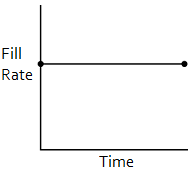D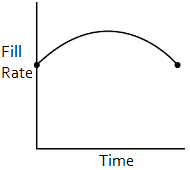Question 2 Explanation:
For a given fill rate, the height will rise slowly when the container is wide and quickly when the container is narrow. To have the height of water rise at a constant rate, we need to decrease the fill rate as the container narrows and increase it as it widens. Only answer (A) meets these requirements.
 Question 3

### A cylindrical birthday cake with a height of 4 inches is cut into two pieces such that each piece is of a different size. If the ratio of the volume of the larger slice to the volume of the smaller slice is 5 to 3, what is the degree measure of the cut made into the cake?

 A 115° B 120° C 135° D 145°
Question 3 Explanation:
Since the ratio of the larger slice to the smaller slice is 5 to 3, the ratio of the area of the smaller slice to the area of the entire cake must be 3 to 8.

This ratio is the same as the ratio of the interior angle of the smaller slice to 360° (the entire cake).

We can therefore set up a proportion:

$\dfrac{3}{8} = \dfrac{x}{360}$

$360(3) = 8x$

$1080 = 8x$

$135 = x$
 Question 4

### On a coordinate plane, ($a$, $b$) and ($a + 5$, $b + c$), and ($13$, $10$) are three points on line $l$. If the $x$-intercept of line $l$ is $−7$, what is the value of $c$?

 A 1.5 B 2 C 2.5 D 3
Question 4 Explanation:
Recall that all lines can be written in the form $y = mx + b$, where $m$ is the slope of the line (rise/run), and $b$ is the $y$-intercept of the line.

Given that the coordinate plane uses the variables $a$ and $b$ for $x$ and $y$, we can rewrite the line equation as: $b = ma + k$, where the variable $k$ is used to replace the original variable $b$ for the $y$-intercept to avoid confusion.

Using this revised equation in conjunction with the given points, we can first solve for the slope of the line in terms of $c$. Given that the slope of a line is the change in the $y$ variable divided by the change in the $x$ variable, calculate the line’s slope:

$\text{slope} = \dfrac{y_2 − y_1}{x_2 − x_1}$

$= \dfrac{(b + c) − b}{(a + 5) − a} = \dfrac{c}{5}$

Substitute this value for the slope into the linear equation:

$b = \dfrac{c}{5} \ast a + k$

Notice that the question only asks for the value of $c$, which is a part of the slope of the line. If we use the given information to determine the actual value of the slope, we can equate the expression containing $c$ with the actual value and solve for $c$.

Given that the line has an x-intercept of −7, we can deduce that (−7, 0) is a point on the line. Calculate the slope of the line using this point and the point (13,10):

$\text{slope} = \dfrac{10 − 0}{13 − (−7)}$

$= \dfrac{10}{20} = \dfrac{1}{2}$

Equate this value with the expression containing $c$:

$\dfrac{1}{2} = \dfrac{c}{5}$

$c = 5 \ast \dfrac{1}{2} = 2.5$
 Question 5

### Under which conditions is the expression $\dfrac{ab}{a − b}$ always less than zero?

 A $a \lt b \lt 0$ B $0 \lt b \lt a$ C $a \lt 0 \lt b$ D $b \lt a \lt 0$
Question 5 Explanation:
This is an example of a problem where it may be simplest to work backwards (check each of the answers to see which one works). For each of the answers, choose values for $a$ and $b$ that meet the criteria then plug these values into the original expression to see if they satisfy the requirement.

(A) $a < b < 0:$

Let $a= -2$ and $b= -1$

$→ \frac{[(-2)(-1)]}{[-2 - (-1)]} = \frac{2}{-1} = -2$ (works)

(Note: this makes sense since if $a$ and $b$ are both negative (less than zero), their product will be positive; and if $a$ is less than $b$, then $a − b$ will be negative; thus, we will have a positive numerator and a negative denominator, resulting in a negative quotient.)

(B) $0 < b < a:$

Let $b=1$ and $a=2$

$→ \frac{[(2)(1)]}{[2 - 1]} = \frac{2}{1} = 2$ (does not work)

(C) $a < 0 < b:$

Let $a=-1$ and $b=1$

$→ \frac{[(-1)(1)]}{[(-1) - (1)]} = \frac{-1}{-2} = \frac{1}{2}$ (does not work)

(D) $b < a < 0:$

Let $b=-2$ and $a=-1$

$→ \frac{[(-1)(-2)]}{[-1 - (-2)]} = \frac{2}{1} = 2$ (does not work)
 Question 6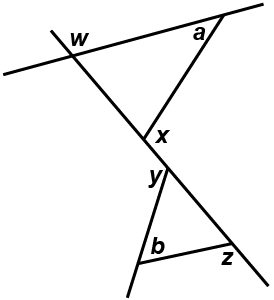### What is the value of $a + b \,$?

 A $w − x − xy + z$ B $360 − x + y + w + z$ C $180 − y + z − w$ D $w + x + y + z − 360$
Question 6 Explanation:
Recall that angles forming a straight line and the 3 interior angles of a triangle each sum to 180°. Using these relationships and substituting expressions for the unlabeled angles, we can then solve the equations in terms of $a$ and $b$. We can then combine these equations to solve for $a + b$.

For the top triangle:
$a + (180 − w) + (180 − x)$ $= 180$°
$360 + a − w − x = 180$
$a = −180 + w + x$

For the bottom triangle:
$b + (180 − y) + (180 − z)$ $= 180$°
$360 + b − y − z = 180$
$b = −180 + y + z$

$a + b = (−180 + w + x)$ $+ \, (−180 + y + z)$
$a + b = −360 + w + x$ $+ \, y + z$
 Question 7

### A sphere with a diameter of 5 cm is being machined out of a cube that is just large enough to contain the inscribed sphere. If the sphere weighs 52 grams, which of the following best approximates the weight of material removed from the cube during the machining of the sphere?

Note: The volume of a sphere $= \left(\frac{4}{3}\right) \pi \, r^3$

 A 75 g B 54 g C 60 g D 47 g
Question 7 Explanation:
The volume of material removed during the machining will equal the volume of the cube minus the volume of the sphere.

The volume of the cube:

$=\text{length} \ast \text{width} \ast \text{height}$
$= 5 \ast 5 \ast 5 = 125 \text{ cm}^3$

The volume of the sphere:

$= \frac{4}{3} \pi (\frac{5}{2})^3 ≈ 65.44 \text{ cm}^3$

So the volume of the material removed from the cube is:

$125 − 65.44 ≈ 59.55 \text{ cm}^3$

The weight of the removed material can be found using a proportion:

$\dfrac{52 \text{ g}}{65.44 \text{ cm}^3} = \dfrac{x}{59.55 \text{ cm}^3}$

$x = 47.32 \text{ g} ≈ 47 \text{ g}$
 Question 8

### Parallelogram QRST has an area of 120 and its longest side (QT) is 24. The angle opposite the vertical is 30°, and the vertical is from R to point U, which lies along QT. What is the length of the hypotenuse of Triangle QRU, rounded to the nearest whole number?A 6 B 8 C 10 D 13
Question 8 Explanation:
Since the area is 120 and the base is 24, we know that the height (RU) must be 5.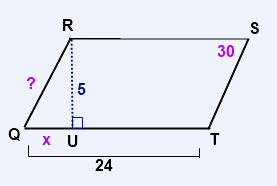Given that the angle opposite the vertical is 30°, we can observe a 30°, 60°, 90° triangle.

Recall that the ratio of the side lengths of a 30°, 60°, 90° triangle is:
$x:x\sqrt{3}:2x$

In this case, the smallest side length $x$ is 5, so:
$5:5\sqrt{3}:2*5$

The hypotenuse is: $2 * 5 = 10$
 Question 9

### A pool that holds 35,000 cubic feet of water is being filled by a pump at a rate of 200 cubic feet per minute. At the same time, water is draining out through an open valve accidentally left open. If the pool is full in 200 minutes, how many cubic feet of water are draining out per minute?

 A 5 B 15 C 25 D 35
Question 9 Explanation:
Write an equation to model the situation, using the rates per minute and the duration of 200 minutes:

$\dfrac{200 \text{ ft}^3}{\text{min}} \ast 200 − \dfrac{x \text{ ft}^3}{\text{min}} \ast 200$ $= 35{,}000 \text{ ft}^3$

Factoring out 200:

$200(200 - x) = 35,000$

Divide by 200:

$200 - x = 175$

$x = 25$ $\dfrac{\text{ ft}^3}{\text{min}}$
 Question 10

### A movie theater charges \$11.50 for an Adult ticket and \$9.75 for a Child ticket. The theater offers a 20% discount on all tickets whenever 10 or more tickets are purchased together. Brenda spent \$95.60 total buying tickets for her group. Seven (7) of the tickets she purchased were Adult tickets. How many Child tickets did she purchase?  A 2 B 1 C 4 D 3 Question 10 Explanation: We are not sure whether she purchased less than 10 tickets with no discount or 10 or more with the 20% discount: Case 1 — Less than 10 tickets 7(\$11.50) + $c$ (\$9.75) = \$95.60
Solving for $c$ we find $c$ = 1.5 tickets. This does not make sense since one cannot purchase half a ticket.

Case 2 — 10 tickets or more
(\$11.50) (1 − 0.20) = \$9.20
Child ticket with 20% discount:
(\$9.75) (1 − 0.20) = \$7.80

7(\$9.20) +$c$(\$7.80) = \$95.60 Solving for$c$,$c$= 4 tickets.  Question 11 ### The circumference of a right cylinder is half its height. The radius of the cylinder is$x$. What is the volume of the cylinder in terms of$x$?  A$2 \pi x^3$B$3 \pi x^3$C$3\pi^2x^3$D$4\pi^2x^3$Question 11 Explanation: Begin by noting the relevant formulas: Circumference:$C=2 \pi r$Volume:$V = \pi r^2 h$Because the radius is equal to$x$, and the circumference is equal to half the height, we can write:$C = 2 \pi r = \frac{1}{2} h$, or$h = 4 \pi r$Substitute this expression for$h$into the volume formula:$V = \pi x^2 (4 \pi x) = 4 \pi^2x^2$ Question 12 ### In the given figure, the measure of angle OAC is 60 degrees, and the center of the circle is O. If the circle has a radius of 6, what is the length of segment DB?A$3$B$3\sqrt{2}$C$6$D$6\sqrt{2}$Question 12 Explanation: Since O is the center, AO = DO = CO = BO = 6. This means that both triangles are isosceles. If triangle AOC is isosceles, and angle ∠OAC = 60°, then angle ∠ACO = 60°. This leaves 180° − 120° = 60° degrees for the third angle ∠AOC. Triangle AOC = equilateral, and angle ∠DOB is vertical with angle ∠AOC and will also equal 60 degrees. Because DO = BO, angle ∠OBD = ∠ODB. To solve for angle ∠OBD: 180° - 2x = 60°, so x = 60°. All three angles = 60°, so therefore, triangle DOB will also be equilateral. Length of DB = 6, which is choice (C).  Question 13 ### Populations of Groups A and B can be modeled exponentially. The increasing population of Group A is described by: ###$P_A = 100e^{.02t}$### The decreasing population of Group B is described by: ###$P_B = 2000e^{−.01t}$### In both equations$t$represents time in minutes. At what time (in minutes) will the Group A population be twice that of Group B?  A 77 B 100 C 123 D 146 Question 13 Explanation: Start by using an equation that shows$P_A$being twice$P_B$:$P_A = 2P_B$Substitute in the given equations:$100e^{.02t} = 2(2000e^{−.01t})$Either use the solver on your calculator or solve algebraically:$100e^{.02t} = 4000e^{−.01t}e^{.02t} = 40e^{−.01t}\ln(e^{.02t}) = \ln(40e^{−.01t})\ln(e^{.02t}) = \ln40 + ln(e^{−.01t}).02t = \ln40 + (−.01t).03t = \ln40t = \dfrac{\ln40}{.03}t = 122.96 ≈ 123 \text{ minutes}$ Question 14 ### A company uses an additive manufacturing (“3D printing”) process to create square pyramids shown in Figure A. What will be the height (rounded to the nearest hundredth) of the object shown in Figure B when half of the material required to print a complete pyramid has been used (“printed”)?A 0.62 cm B 1.50 cm C 1.33 cm D 0.75 cm Question 14 Explanation: The simplest approach is to realize that if half the material has been used, then half of the material remains to be used. Thus, there is an unprinted pyramid at the top that still needs to be printed. How large will this unprinted pyramid be? The key is to realize that the base dimensions will be the same as the height (altitude) of our unprinted pyramid.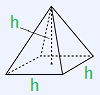$V_{Half} = \frac{1}{2}(\frac{1}{3}(3 \cdot 3 \cdot 3))= 4.5 \text{ cm}^3V_{Unprinted} = \frac{1}{3}(h \cdot h \cdot h)= 4.5 \text{ cm}^3$Solving for$h$, gives$h = 2.38 \text{ cm}$. Since the total height of the full pyramid is$3 \text{ cm}$, then the height of the printed portion is$3 − 2.38 \text{ cm} = 0.62 \text{ cm}$.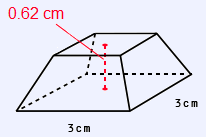Question 15 ### Line F is represented by the equation$y = x + 1$. Line G is represented by the equation$y = px + q$. Line F and Line G always intersects where$x = 1$. What equation properly expresses the relationship between$p$and$q \,$?  A$p = −q + 2$B$p = q − 2$C$p = 2q + 1$D$p = 2q − 1$Question 15 Explanation: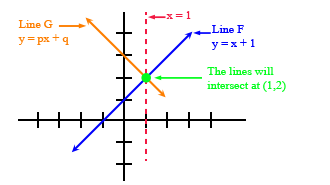We are told that Line F and Line G intersect where$x = 1$. We can find the$y$value when$x = 1$, by using the equation for Line F:$y = x + 1$(given)$y = 1 + 1$(substituting$1$for$x$)$y = 2$This means that the lines intersect at the point ($1, 2$); both lines contain this point. The equation for Line G is:$y = px + q$Substituting in$1$for$x$and$2$for$y$we have:$2 = p(1) + q$Solving for$p$yields:$p = 2 − q$or$p = −q + 2\$
Once you are finished, click the button below. Any items you have not completed will be marked incorrect.
There are 15 questions to complete.
 ← List →